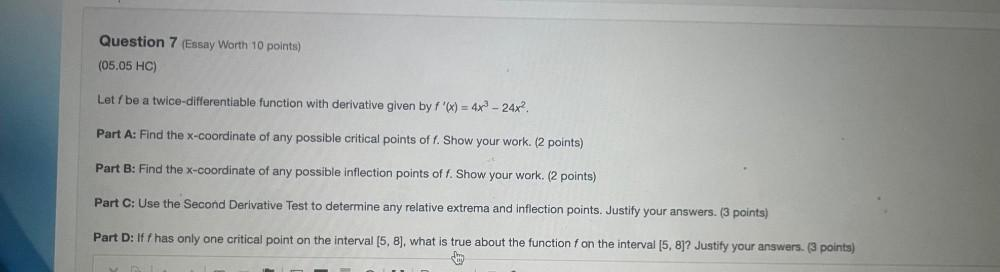Question:

# Let f be a twice-differentiable function with derivative given by f '(x) = 4x^3 - 24x^2. A): Find the x-coordinate of any possible critical points of f. Show your work. B): Find the x-coordinate oLet f be a twice-differentiable function with derivative given by f '(x) = 4x^3 - 24x^2. A): Find the x-coordinate of any possible critical points of f. Show your work. B): Find the x-coordinate of any possible inflection points of f. Show your work. C): Use the Second Derivative Test to determine any relative extrema and inflection points. Justify your answers. D): If f has only one critical point on the interval [5, 8], what is true about the function f on the interval [5, 8]? Justify your answers.